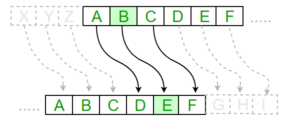# Substitution Cipher

## Substitution Cipher

Hiding some data is known as encryption. When plain text is encrypted it becomes unreadable and is known as ciphertext. In a Substitution cipher, any character of plain text from the given fixed set of characters is substituted by some other character from the same set depending on a key. For example with a shift of 1, A would be replaced by B, B would become C, and so on.

Note: Special case of Substitution cipher is known as Caesar cipher where the key is taken as 3.

#### Mathematical representation

The encryption can be represented using modular arithmetic by first transforming the letters into numbers, according to the scheme, A = 0, B = 1,…, Z = 25. Encryption of a letter by a shift n can be described mathematically as.(Encryption Phase with shift n)(Decryption Phase with shift n)Examples:

```Plain Text: I am studying Data Encryption
Key: 4
Output: M eq wxyhCmrk Hexe IrgvCtxmsr

Plain Text: ABCDEFGHIJKLMNOPQRSTUVWXYZ
Key: 4
Output: EFGHIJKLMNOPQRSTUVWXYZabcd```

Algorithm for Substitution Cipher:

Input:

• A String of both lower and upper case letters, called PlainText.
• An Integer denoting the required key.

Procedure:

• Create a list of all the characters.
• Create a dictionary to store the substitution for all characters.
• For each character, transform the given character as per the rule, depending on whether we’re encrypting or decrypting the text.
• Print the new string generated.

Below is the implementation.

 `# Python program to demonstrate` `# Substitution Cipher` `import` `string` `# A list containing all characters` `all_letters``=` `string.ascii_letters` ` ` `    ` `"""` `create a dictionary to store the substitution` `for the given alphabet in the plain text` `based on the key` `"""` ` ` `    ` `dict1 ``=` `{}` `key ``=` `4` ` ` `for` `i ``in` `range``(``len``(all_letters)):` `    ``dict1[all_letters[i]] ``=` `all_letters[(i``+``key)``%``len``(all_letters)]` ` ` ` ` `plain_txt``=` `"I am studying Data Encryption"` `cipher_txt``=``[]` ` ` `# loop to generate ciphertext` ` ` `for` `char ``in` `plain_txt:` `    ``if` `char ``in` `all_letters:` `        ``temp ``=` `dict1[char]` `        ``cipher_txt.append(temp)` `    ``else``:` `        ``temp ``=``char` `        ``cipher_txt.append(temp)` `        ` `cipher_txt``=` `"".join(cipher_txt)` `print``(``"Cipher Text is: "``,cipher_txt)` ` ` `    ` `"""` `create a dictionary to store the substitution` `for the given alphabet in the cipher` `text based on the key` `"""` ` ` `    ` `dict2 ``=` `{}    ` `for` `i ``in` `range``(``len``(all_letters)):` `    ``dict2[all_letters[i]] ``=` `all_letters[(i``-``key)``%``(``len``(all_letters))]` `     ` `# loop to recover plain text` `decrypt_txt ``=` `[]` `for` `char ``in` `cipher_txt:` `    ``if` `char ``in` `all_letters:` `        ``temp ``=` `dict2[char]` `        ``decrypt_txt.append(temp)` `    ``else``:` `        ``temp ``=` `char` `        ``decrypt_txt.append(temp)` `        ` `decrypt_txt ``=` `"".join(decrypt_txt)` `print``(``"Recovered plain text :"``, decrypt_txt)`

Output:

```Cipher Text is:  M eq wxyhCmrk Hexe IrgvCtxmsr
Recovered plain text : I am studying Data Encryption```

Last Updated on November 13, 2021 by admin

## Sort Correlation Matrix in PythonSort Correlation Matrix in Python

Sort Correlation Matrix in Python A correlation matrix investigates the dependence between multiple variables at

## Creating a Pandas SeriesCreating a Pandas Series

Pandas Series is a one-dimensional labelled array capable of holding data of any type (integer,

## How to Install Python Pandas on Windows and Linux?How to Install Python Pandas on Windows and Linux?

Install Python Pandas on Windows and Linux   Pandas in Python is a package that

## Python program to find smallest number in a listPython program to find smallest number in a list

Given a list of numbers, the task is to write a Python program to find

## Python – Convert dictionary object into stringPython – Convert dictionary object into string

Python | Convert dictionary object into string Dictionary is an important container and used almost

## Indexing and Selecting Data with PandasIndexing and Selecting Data with Pandas

Indexing and Selecting Data with Pandas Indexing in Pandas : Indexing in pandas means simply

## Python program to create a dictionary from a stringPython program to create a dictionary from a string

Python program to create a dictionary from a string Dictionary in python is a very useful

## Python – Output FormattingPython – Output Formatting

Python – Output Formatting There are several ways to present the output of a program,